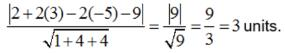# Test: Three Dimensional Geometry (CBSE Level) - 2

## 25 Questions MCQ Test Mathematics (Maths) Class 12 | Test: Three Dimensional Geometry (CBSE Level) - 2

Description
This mock test of Test: Three Dimensional Geometry (CBSE Level) - 2 for JEE helps you for every JEE entrance exam. This contains 25 Multiple Choice Questions for JEE Test: Three Dimensional Geometry (CBSE Level) - 2 (mcq) to study with solutions a complete question bank. The solved questions answers in this Test: Three Dimensional Geometry (CBSE Level) - 2 quiz give you a good mix of easy questions and tough questions. JEE students definitely take this Test: Three Dimensional Geometry (CBSE Level) - 2 exercise for a better result in the exam. You can find other Test: Three Dimensional Geometry (CBSE Level) - 2 extra questions, long questions & short questions for JEE on EduRev as well by searching above.
QUESTION: 1

### Skew lines are lines in different planes which are

Solution:

By definition : The Skew lines are lines in different planes which are neither parallel nor intersecting .

QUESTION: 2

### If a line has the direction ratios – 18, 12, – 4, then what are its direction cosines ?

Solution:

If a line has the direction ratios – 18, 12, – 4, then its direction cosines are given by: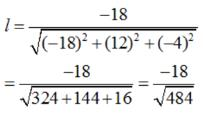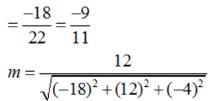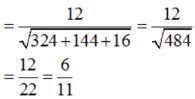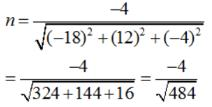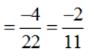QUESTION: 3

### Equation of a plane which is at a distance d from the origin and the direction cosines of the normal to the plane are l, m, n is.

Solution:

In Cartesian co – ordinate system Equation of a plane which is at a distance d from the origin and the direction cosines of the normal to the plane are l, m, n is given by : lx + my + nz = d.

QUESTION: 4

Find the vector equation of a plane which is at a distance of 7 units from the origin and normal to the vector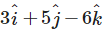Solution: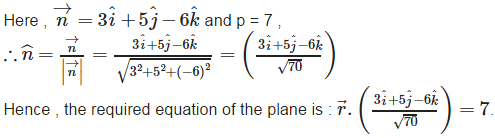QUESTION: 5

In the following case, determine whether the given planes are parallel or perpendicular, and in case they are neither, find the angles between them. 2x – y + 3z – 1 = 0 and 2x – y + 3z + 3 = 0

Solution: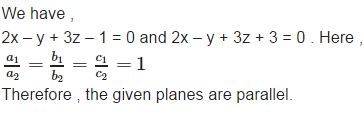QUESTION: 6

Angle between skew lines is

Solution:

Angle between skew lines is the angle between two intersecting lines drawn from any point

QUESTION: 7

Find the equation of the line which passes through the point (1, 2, 3) and is parallel to the vector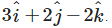Solution:

The equation of the line which passes through the point (1, 2, 3) and is parallel to the vector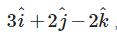Let vector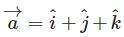and vector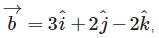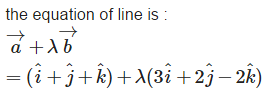QUESTION: 8

The equation of a plane through a point whose position vector is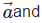perpendicular to the vector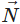. is

Solution:

In vector form The equation of a plane through a point whose position vector isperpendicular to the vectorIs given by :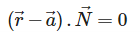QUESTION: 9

Find the Cartesian equation of the plane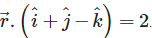Solution: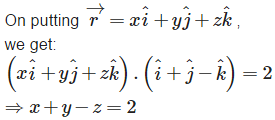QUESTION: 10

In the following case, determine whether the given planes are parallel or perpendicular, and in case they are neither, find the angles between them. 4x + 8y + z – 8 = 0 and y + z – 4 = 0

Solution:

We have, 4x + 8y + z – 8 = 0 and y + z – 4 = 0. Let be the angle between the planes, then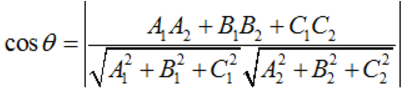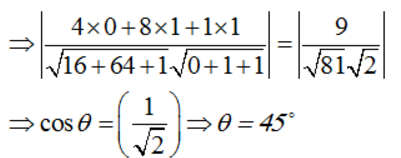QUESTION: 11

If l1, m1, n1 and l2, m2, n2 are the direction cosines of two lines; and θ is the acute angle between the two lines; then

Solution:

If l1, m1, n1 and l2, m2, n2 are the direction cosines of two lines; and θ is the acute angle between the two lines; then the cosine of the angle between these two lines is given by :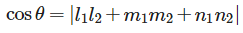QUESTION: 12

Find the equation of the line in cartesian form that passes through the point with position vector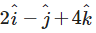and is in the direction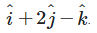Solution: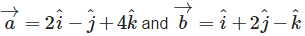, then , its Cartesian equation is given by :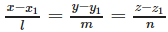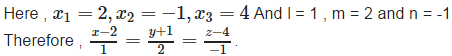QUESTION: 13

Equation of a plane passing through three non collinear points (x1, y1, z1),(x2, y2, z2) and (x3, y3, z3) is

Solution:

In cartesian co – ordinate system : Equation of a plane passing through three non collinear points (x1, y1, z1),(x2, y2, z2) and (x3, y3, z3) is given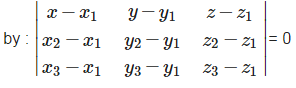QUESTION: 14

Find the Cartesian equation of the plane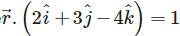Solution: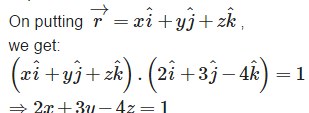QUESTION: 15

Find the distance of the point (0, 0, 0) from the plane 3x – 4y + 12 z = 3

Solution:

As we know that the length of the perpendicular from point
P(x1,y1,z1) from the plane a1x+b1y+c1z+d1 = 0 is given by: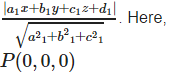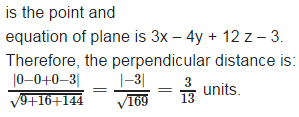QUESTION: 16

If a1, b1, c1 and a2, b2, c2 are the direction ratios of two lines and θ is the acute angle between the two lines; then

Solution:

If a1, b1, c1 and a2, b2, c2 are the direction ratios of two lines and θθ is the acute angle between the two lines; then , the cosine of the angle between these two lines is given by :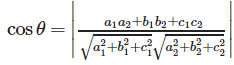QUESTION: 17

Find the equation of the line in cartesian form that passes through the point (– 2, 4, – 5) and parallel to the line given by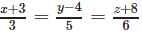Solution:

Find the equation of the line in cartesian form that passes through the point (– 2, 4, – 5) and parallel to the line given by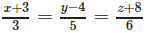is given by: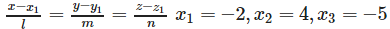And l = 3 , m = 5 and n = 6 .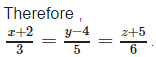QUESTION: 18

Vector equation of a plane that contains three non collinear points having position vectors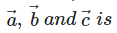Solution:

Vector equation of a plane that contains three non collinear points having position vectors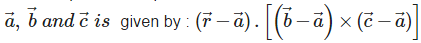QUESTION: 19

The vector and cartesian equations of the planes that passes through the point (1, 0, – 2) and the normal to the plane is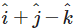Solution:

Let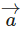be the position vector of the point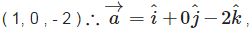here,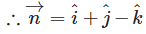. Therefore, the required vector equation of the plane is: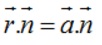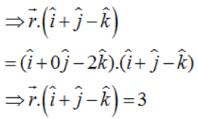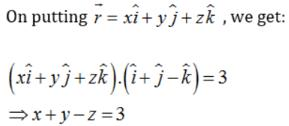QUESTION: 20

Find the distance of the point (3, – 2, 1) from the plane 2x – y + 2z + 3 = 0

Solution:

As we know that the length of the perpendicular from point P(x1,y1,z1) from the plane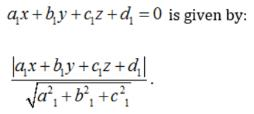Here, P(3, - 2,1) is the point and equation of Plane is 2x - y + 2z+3 = 0

Therefore, the perpendicular distance is :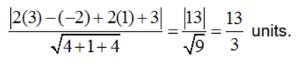QUESTION: 21

Vector equation of a line that passes through the given point whose position vector is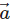and parallel to a given vector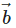is

Solution:

Vector equation of a line that passes through the given point whose position vector isand parallel to a given vectoris given by :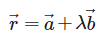QUESTION: 22

Find the values of p so that the lines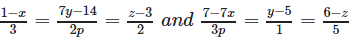are at right angles.

Solution:

Give lines are :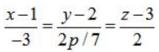and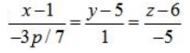The D.R's of the lines are -3, 2p/7, 2 and -3p/7, 1, -5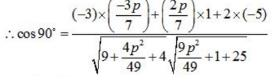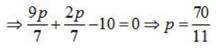QUESTION: 23

Vector equation of a plane that passes through the intersection of planes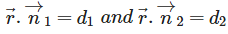expressed in terms of a non – zero constant λ is

Solution:

In vector form:
Vector equation of a plane that passes through the intersection of planes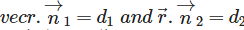expressed in terms of a non – zero constant λ is given by :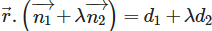QUESTION: 24

Find the equations of the planes that passes through three points (1, 1, 0), (1, 2, 1), (– 2, 2, – 1)

Solution:

In cartesian co-ordinate system :
Equation of a plane passing through three non collinear

Points (x1, y1, z1) , (x2, y2, z2) and (x3, y3, z3) is given by :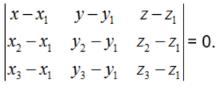Therefore, the equations of the planes that passes through three points (1,1,0), (1,2,1),  (-2,2,-1) is given by :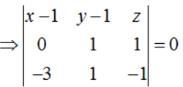⇒ (x-1)(-2) - (y-1) (3) + 3z = 0
⇒ 2x+3y - 3z = 5

QUESTION: 25

Find the distance of the point (2, 3, – 5) from the plane x + 2y – 2z = 9

Solution:

As we know that the length of the perpendicular from point P(x1,y1,z1) from the plane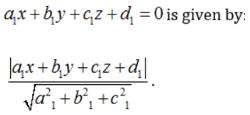Here, P(2,3,-5) is the point and equation of plane is x+2y - 2z = 9

Therefore, the perpendicular distance is :